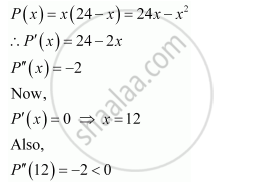Share

# Find Two Numbers Whose Sum is 24 and Whose Product is as Large as Possible. - CBSE (Commerce) Class 12 - Mathematics

#### Question

Find two numbers whose sum is 24 and whose product is as large as possible.

#### Solution

Let one number be x. Then, the other number is (24 − x).

Let P(x) denote the product of the two numbers. Thus, we have:∴By second derivative test, x = 12 is the point of local maxima of P. Hence, the product of the numbers is the maximum when the numbers are 12 and 24 − 12 = 12

Is there an error in this question or solution?

#### APPEARS IN

NCERT Solution for Mathematics Textbook for Class 12 (2018 to Current)
Chapter 6: Application of Derivatives
Q: 13 | Page no. 233

#### Video TutorialsVIEW ALL 

Solution Find Two Numbers Whose Sum is 24 and Whose Product is as Large as Possible. Concept: Maxima and Minima.
S